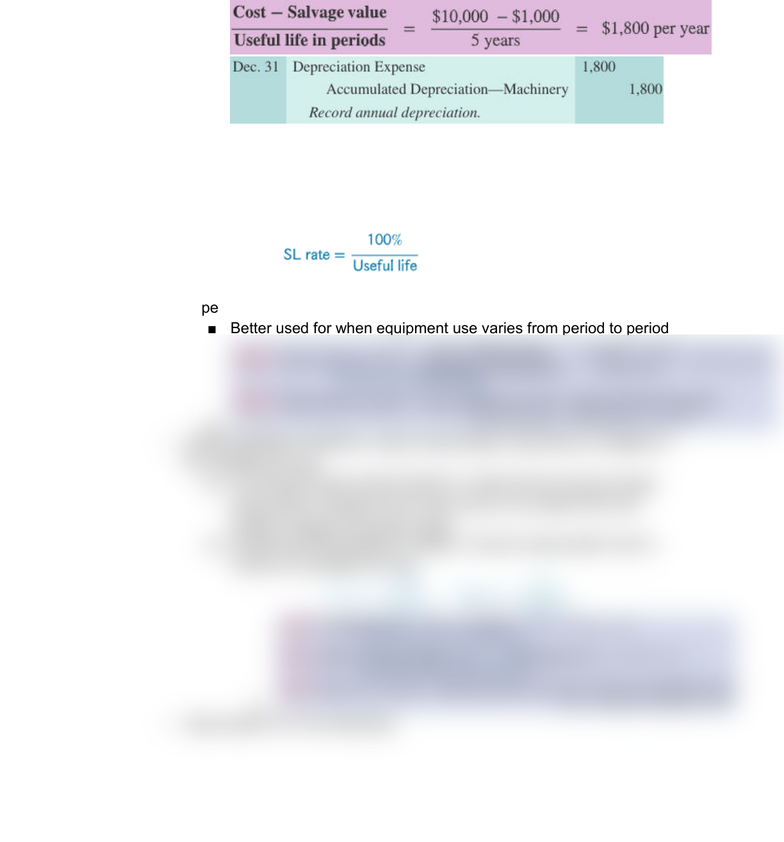Textbook Notes (280,000)
US (110,000)
UC-Davis (1,000)
MGT 11A (30)
Chapter 8

# MGT 11A Chapter Notes - Chapter 8: Ddb Worldwide, Macrs, Financial Statement

Department
Management
Course Code
MGT 11A
Professor
John Hancock
Chapter
8

This preview shows half of the first page. to view the full 2 pages of the document.DEPRECIATION
Depreciation Methods:
Straight-Line Method: charge the same amount to each period of the
asset’s useful life
Asset Book Value: Asset’s total cost - Accumulated Depreciation
Ex: \$10,000 - (2 yrs x \$1,800) = book value
Straight-line Depreciation Rate:
100% divided by the number of periods in the asset’s useful
life
Units-of-Production Method: charge a varying amount for each period
depending on asset’s usage
Better used for when equipment use varies from period to period
Declining-Balance Method: uses a depreciation rate that is a multiple of
the straight-line rate
Accelerated Depreciation Method: method that produces larger
depreciation charges in the early years of an asset’s life and
smaller charged in its later years
Double-Declining Balance (DDB): common depreciation rate is
double the straight-line rate
Depreciation for Tax Reporting